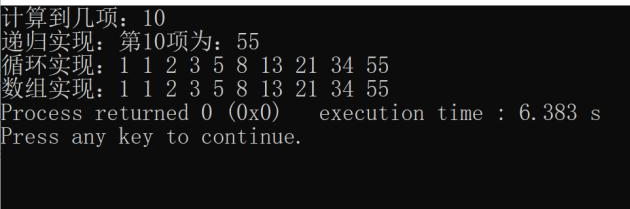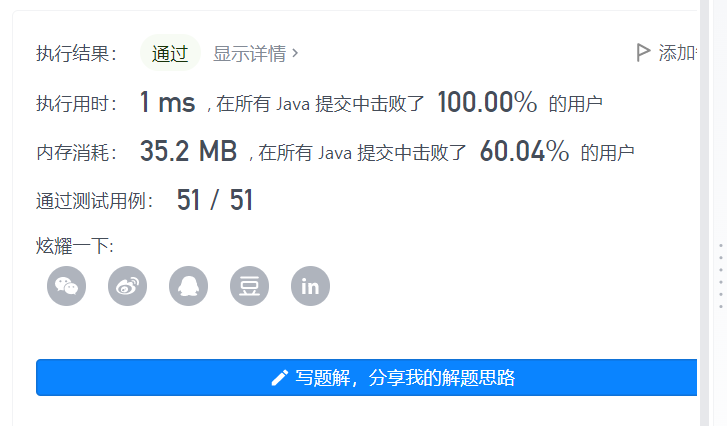• 2020-09-11 10:57:31
/**
* 斐波那契函数的递归实现
*
* 1 1 2 3 5 8 13 21
*/
public class FibonacciTest {

private int []data;

public FibonacciTest(int n) {
data = new int[n];
}

public static void main(String[] args) {
FibonacciTest fib = new FibonacciTest(100);
long startTime = System.currentTimeMillis();
System.out.println(fib.fibonacci_1(30));
long endTime = System.currentTimeMillis();
System.out.println("fibonacci_1 cost " + (endTime - startTime) + "ms");
System.out.println(fib.fibonacci_2(30));
long endTime1 = System.currentTimeMillis();
System.out.println("fibonacci_2 cost " + (endTime1 - endTime) + "ms");
System.out.println(fib.fibonacci_3(1,1,30));
long endTime2 = System.currentTimeMillis();
System.out.println("fibonacci_3 cost " + (endTime2 - endTime1) + "ms");
}

/**
* 递归函数，时间复杂度O(n^2)
* @param n
* @return
*/
public int fibonacci_1(int n) {
if (n <= 2) {
return 1;
}
return fibonacci_1(n-1) + fibonacci_1(n-2);
}

/**
* 采用缓存进行递归 时间复杂度O(n)
* @param n
* @return
*/
public int fibonacci_2(int n) {
if (n <= 2) {
return 1;
}
int num = data[n];
if (num > 0)
return num;
int res =  fibonacci_2(n-1) + fibonacci_2(n-2);
data[n] = res;
return res;
}

/**
* 采用尾递归 时间复杂度O(n)
* n的长度需要大于2
* 计算是从前往后累加
* @param n
* @return
*/
public int fibonacci_3(int pre, int pre_next, int n) {
if (n <= 2) {
return pre;
}
int new_pre_next = pre;
int new_pre = pre_next +pre;
return fibonacci_3(new_pre, new_pre_next, n-1);
}

}算法 java
更多相关内容
• //斐波那契 三种方法： int x = Fib_1(6); int y = Fib_2(7); int z = Fib_3(8); 方法实现： #region 斐波那契计算 递推公式：F(n)=F(n-1)+F(n-2) /// <summary> ///递归方式 性能不好 1、1、2、3、5...

//斐波那契 三种方法：
int x = Fib_1(6);
int y = Fib_2(7);
int z = Fib_3(8);

方法实现：

#region 斐波那契计算 递推公式：F(n)=F(n-1)+F(n-2)
/// <summary>
///递归方式 性能不好 1、1、2、3、5、8、13、21、34、……，这个数列从第3项开始，每一项都等于前两项之和。求第n个数是几
/// </summary>
/// <param name="n">第n个数,下标从1开始</param>
/// <returns></returns>
static int Fib_1(int n)
{
if (n <= 0)
return 0;
else if (n >= 1 && n <= 2)
return 1;
else
return Fib_1(n - 1) + Fib_1(n - 2);
}
/// <summary>
/// 数组方式 性能高于上面的递归
/// </summary>
/// <param name="n"></param>
/// <returns></returns>
static int Fib_2(int n)
{
if (n <= 0)
{
return 0;
}
else if (n >= 1 && n <= 2)
{
return 1;
}
else
{
int[] arr = new int[n];
arr = 1;
arr = 1;
for (int i = 2; i < n; i++)
{
arr[i] = arr[i - 1] + arr[i - 2];
}
return arr[n - 1];
}
}
/// <summary>
/// 循环方式 性能高于上面的数组
/// </summary>
/// <param name="n"></param>
/// <returns></returns>
static int Fib_3(int n)
{
int result = 0;
int last = 1;
int lastnext = 1;

for (int x = 2; x < n; x++)
{
result = last + lastnext;
lastnext = last;
last = result;
}

return result;
}
#endregion

展开全文c# 开发语言
• 斐波那契数列的三种算法，算法一：递归 #include #include long int fibo(int n) { if(n==0) return 0; if(n==1) return 1; return fibo(n-1)+fibo(n-2); } void main() {
• 二、斐波那契数列的三种实现方法1.递归2.简单循环，无数组3.数组三、完整代码 前言 C++ 得到斐波那契数列三种方法 一、斐波那契数列是什么？ 斐波那契数列（Fibonacci sequence） 又称黄金分割数列，因数学家...

# 前言

C++ 得到斐波那契数列三种方法

# 一、斐波那契数列是什么？

斐波那契数列（Fibonacci sequence）
又称黄金分割数列，因数学家莱昂纳多·斐波那契（Leonardoda Fibonacci）以兔子繁殖为例子而引入，故又称为“兔子数列”。

指的是这样一个数列：0、1、1、2、3、5、8、13、21、34、……
在数学上，斐波那契数列以如下被以递推的方法定义：F(0)=0，F(1)=1, F(n)=F(n - 1)+F(n - 2)（n ≥ 2，n ∈ N*）

# 二、斐波那契数列的三种实现方法

## 1.递归

代码如下（示例）：

//递归方法
int Recursion(int n)
{
if(n<=2) return 1;
return Recursion(n-1)+Recursion(n-2);
}



## 2.简单循环，无数组

代码如下（示例）：

//简单循环，无数组
int Loop(int n)
{
int f,b,temp;
f=0;
b=1;
if(n<=1) return 1;
for(int i=1;i<n;i++)
{
printf("%d ",b);
temp=f+b;
f=b;
b=temp;
}
}



## 3.数组

代码如下（示例）：

//数组
int Array(int n)
{
int a[max];
a=0;a=1;
if(n>1)
{
for(int i=2;i<=n;i++) a[i]=a[i-1]+a[i-2];
}
}


# 三、完整代码

#include <iostream>
#include <stdio.h>
#define max 10005
using namespace std;

//递归
int Recursion(int n)
{
if(n<=2) return 1;
return Recursion(n-1)+Recursion(n-2);
}

//简单循环，无数组
int Loop(int n)
{
int f,b,temp;
f=0;
b=1;
if(n<=1) return 1;
for(int i=1;i<n;i++)
{
printf("%d ",b);
temp=f+b;
f=b;
b=temp;
}
//打印输出
printf("%d",b);
}

//数组
int Array(int n)
{
int a[max];
a=0;a=1;
if(n>1)
{
for(int i=2;i<=n;i++) a[i]=a[i-1]+a[i-2];
}
//打印输出
for(int i=1;i<=n;i++) printf("%d ",a[i]);
}

int main()
{
int n;
printf("计算到几项：");
scanf("%d",&n);

printf("递归实现：");
printf("第%d项为：%d",n,Recursion(n));

printf("\n循环实现：");
Loop(n);

printf("\n数组实现：");
Array(n);

return 0;
}


运行结果:展开全文c++ 算法 递归算法
• ：vector实现时间复杂度是0(n)，时间复杂度是0(1)，就是不知道vector的效率高不高，当然vector有自己的属性会占用资源。四：queue实现当然队列比数组更适合实现斐波那契数列，时间复杂度和空间复杂度和vector一样...
• 本题主要是for循环语句，写法有如下两： 1.输入一个变量确定列表长度，for循环用内置函数range确定循环次数，利用切片方法将列表fib最后两数之和追加到列表中，每循环一次追加一个值 2.for循环用内置函数range确定...
• 写一个函数，输入n，求斐波那契Fibonacci）数列的第n项（即F(N)）。斐波那契数列的定义如下： F(0) = 0, F(1)= 1 F(N) = F(N - 1) + F(N - 2), 其中 N > 1. 斐波那契数列由 0 和 1 开始，之后的斐波那契数...

# 题目描述：

剑指 Offer 10- I. 斐波那契数列

难度简单282

写一个函数，输入 n ，求斐波那契（Fibonacci）数列的第 n 项（即 F(N)）。斐波那契数列的定义如下：

F(0) = 0,   F(1) = 1
F(N) = F(N - 1) + F(N - 2), 其中 N > 1.

斐波那契数列由 0 和 1 开始，之后的斐波那契数就是由之前的两数相加而得出。

答案需要取模 1e9+7（1000000007），如计算初始结果为：1000000008，请返回 1。

示例 1：

输入：n = 2
输出：1


示例 2：

输入：n = 5
输出：5


提示：

• 0 <= n <= 100

通过次数298,480提交次数826,785

题目链接：力扣

# 3种解法：

## 一，动态规划解法：

/**
* 斐波那契数列——动态规划解法：
* 时间复杂度为O(n)
* @param n
* @return
*/
public static int fib(int n) {
//1，特殊情况处理：
if (n == 0) {
return 0;
} else if (n == 1) {
return 1;
}
//2，正常情况：
else {
//新建一个数组，用于存放之前的子结果：
int[] array = new int[n + 1];
array = 0;
array = 1;
for (int i = 2; i <= n; i++) {
array[i] = (array[i - 1] + array[i - 2]) % 1000000007;
}
return array[n];
}
}## 二，递归解法：

/**
* 斐波那契数列——递归解法：
* @param n
* @return
*/
public static int fib(int n) {
if (n == 0) {
return 0;
} else if (n == 1) {
return 1;
} else {
n = (fib(n - 1) + fib(n - 2)) % 1000000007;
return n;
}
}

耗时太长。时间复杂度为O(2^n)。

## 三，带备忘录的递归：

private List<Integer> list = new ArrayList<>();
/**
* 斐波那契数列——带备忘录的递归解法：
* 单单使用暴力的递归解法太费时费劲了，时间复杂度为O(2^n)
* @param n
* @return
*/
public static int fib3(int n) {
if (!list.contains(0)) {
}
if (!list.contains(1)) {
}
if (list.size() > n) {
return list.get(n);
} else {
n = (fib3(n - 1) + fib3(n - 2)) % 1000000007;
return n;
}
}直接递归会重复计算，例如fib(4) = fib(3) + fib(2)和fib(3) = fib(2) + fib(1) fib(2)被重复计算。

单单是递归，耗时非常长，时间复杂度为O(2^n)，

改造一下，为带备忘录的递归，存储递归过程中的值，去除重复计算，可以大大减少时间复杂度，优化之后，时间复杂度为O(n)。

展开全文leetcode 算法
• 本篇文章主要介绍了python使用递归、尾递归、循环三种方式实现斐波那契数列，非常具有实用价值，需要的朋友可以参考下 在最开始的时候所有的斐波那契代码都是使用递归的方式来写的，递归有很多的缺点，执行效率低下...
• 本篇文章主要介绍了python使用递归、尾递归、循环三种方式实现斐波那契数列，非常具有实用价值，需要的朋友可以参考下
• 主要为大家详细介绍了三种java编程方法实现斐波那契数列，具有一定的参考价值，感兴趣的小伙伴们可以参考一下
• 斐波那契数列(Fibonacci)JAVA解法 1.递归函数： public class Main { public int f(int n){ if (n == 0 | n==1) return 1; else return (f(n-1)+f(n-2)); } public static void main(String[] args) { ...java 算法
• 斐波那契/ n取参数n并返回斐波那契数列的第n个数字以及以下个数字{Fn，...，Fn + 3} 素数/ Fn + 3从斐波那契结果中获取Fn + 3并返回所有小于或等于该数的素数的列表以供进一步使用{2,3,5，...，P} 解构/ Fn / {2...JavaScript
• 常规的斐波那契数列解法 int fib(int n) { if (n &lt;= 2) { return 1; } else return fib(n - 1) + fib(n - 2); } 要求第n个斐波那契数，子问题就是求每一个斐波那契数的前一项和前二项之和，典型的...
• 斐波那契数列（Fibonacci sequence），又称黄金分割数列、因数学家列昂纳多·斐波那契（Leonardoda Fibonacci）以兔子繁殖为例子而引入，故又称为“兔子数列”，指的是这样一个数列：1、1、2、3、5、8、13、21、...
• Three Way to implement Fibonacci package main import ( "fmt" "time" ) // Fibonacci implemented with definition func Fibonacci(n int) int { if n == 0 { return 0 } if n == 1 { return 1 } ...算法 go语言 开发语言
• 这是一个简单的程序，可用于比较Java中Fibonacci算法的3不同实现，以了解计算时间。 这个实现如下： 递归斐波那契 尾递归斐波那契 迭代斐波那契 结果表明，迭代一次是最快的，而递归一次是最慢的。 因此，递归...Java
• 今天初夏将为大家带来计算斐波那契数列第n位的三种方法 第一种利用递归的方法计算，代码相当简单，但其重复计算率太高，导致其时间复杂度比指数还要爆炸式增长。不推荐此方法。但递归的思想却相当的重要还是要理解...
• 动规是非递归的一代码可以保存下来一些过程的解 1. 把原来的为标题分解成几个相似的子问题 2. 所有的子问题都只需要解决一次 3. 储存子问题的解 动规本质：是对问题状态的定义和状态方程的定义（状态以及状态之间...动态规划 c++ 算法
• 实验内容： ①利用多种方法实现斐波那契数列分别可用循环，递归和分治3种方法，并由此估算三种算法的时间复杂度，比较三种算法的运行效率。 ②首先定义主函数分别定义3个时间变量来作为三种算法的运行时间，并且在...c语言 算法 函数 递归
• 斐波那契数列(Fibonacci sequence)的定义：斐波那契数列指的是这样一个数列 1, 1, 2, 3, 5, 8, 13, 21, 34, 55, 89, 144, 233，377，610，987，1597，2584，4181，6765，10946，17711，28657，46368........，这个...
• 写一个函数，输入 n ，求斐波那契Fibonacci）数列的第 n 项（即 F(N)）。斐波那契数列的定义如下： F(0) = 0, F(1) = 1 F(N) = F(N - 1) + F(N - 2), 其中 N > 1. 斐波那契数列由 0 和 1 开始，之后的斐波那契...c++ leetcode 算法
• dijkstra算法的三种实现：数组，二叉堆，斐波那契堆 + 部分实验报告 dijkstra算法的三种实现：数组，二叉堆，斐波那契堆 + 部分实验报告 dijkstra算法的三种实现：数组，二叉堆，斐波那契堆 + 部分实验报告
• 一、莱昂纳多·斐波那契（Leonardo Fibonacci斐波那契公元1170年生于意大利比萨，卒于1250年，被人称作“比萨的莱昂纳多”。1202年，他撰写了《算盘全书》（Liber Abacci）一书。他是第一个研究了印度和阿拉伯...C# 算法
• 斐波那契数列 0,1,1,2,3,5,8,13,21,34…… f(n)=f(n-1)+f(n-2) f(0)=0 f(1)=1 public static int recursion(int i) { if (i == 0 || i == 1) { return i; } return recursion (i - 1) + recursion (i - 2); ...leetcode 算法 数据结构
• 题目：斐波那契数列，又称黄金分割数列(F(n+1)/F(n)的极限是1:1.618，即黄金分割率)，指的是这样一个数列：0、1、1、2、3、5、8、13、21、34、……。在数学上，斐波纳契数列以如下被以递归的方法定义：F(0)=0，F(1)=......